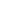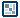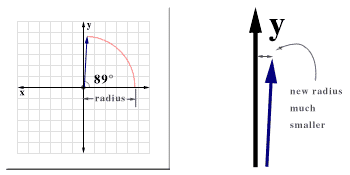## Question of the WeekScripting 3D in Flash       by senocular Trigonometry and Multiple Axes Looking side to side is no problem at all. Moving based on that view is a breeze. Great! Now it's time to throw in another addition to functionality - another rotation. Rotation here won't be around one axis restricted to only one coordinate space as was the case before. Now it's time to not only rotate within x and z to turn left and right, but also z and y to give the impression of being able to look up! It's mostly just a matter of going through the process of rotating along more than one axis, which, at first, may seem simple but this new rotation adds a whole new complication. Sound scary? Good. The good news: This completely plows the way for the next section of discussion (and all that follow) which is fully rotational rendered 3D objects around their centers. The bad news: We'll pretty much need to completely restructure our current approach in rotation and panning. New trig concepts will be introduced here and they will replace some of the already established infrastructure we've been so used to using thus far. Hopefully, though, it should be no big deal. If you've kept up pretty well so far, what's coming up shouldn't be too difficult. And getting past this next step will make everything else a breeze. More Trigonometry - Rotating Around Multiple Axes With 2D rotation around a point, whether it be in a fully 2D space or just within a single plane in a 3D space, you deal primarily with those three principal factors used previously, origin, angle and radius. This origin represents offset or the coordinates of the center of rotation. Angle is the angle from 0 which your rotation is based, somewhere within a 2 PI radian range (360 degrees). And lastly, there's that hypotenuse line length or the radius - the span between the position of the origin and the final rotated point. With those, we got: x = originx + Math.cos(angle)*radius; y = originy + Math.sin(angle)*radius; Rotation using multiple axes is very similar only it needs the same operation above performed more than one time, one for each additional axis being rotated around. Each axis requires their own rotation calculations for the rotation in their own 2D space. The y axis, for example, when rotated around, maintains a position within the x and z 2D plane. If you wanted to rotated an object in complete 3D, you would need to rotate around all 3 axes which would require rotation in each of the planes existing within x, y and z; those being: x and y, x and z, and y and z - each representing a rotation around each axis x y or z. // rotation around x axis y = Math.cos(angleAroundXAxis)*radius; z = Math.sin(angleAroundXAxis)*radius; // rotation around y axis x = Math.cos(angleAroundYAxis)*radius; z = Math.sin(angleAroundYAxis)*radius; // rotation around z axis x = Math.cos(angleAroundZAxis)*radius; y = Math.sin(angleAroundZAxis)*radius; The tricky part here, however, is making them work together. It's not as easy as just placing them in successive order one after the other and just having it work. Don't think that complex 3D would be THAT easy! Just think about what would happen if you wanted to rotate an line around 2 axes, say for example, first around the z, moving in x and y space, and then the y, in x and z space. We start with a line of 0 rotation and a radius of 10. The offset can just be kept at 0,0 since that's not important here and it will make things easier to think about. So let's first rotate around the z and do so at about, oh, 89 degrees. 89 degrees is almost 90 degrees which is straight up. This puts you almost right against, or at least really close, to the y axis itself. And it's the y axis which we'll want to rotate around next. To the y axis, now, however, your radius is no longer 10. Its much, much lower because we've rotated so close to it in rotating around the z axis.[ change in radius size after rotation ] Because of this, you would need to have to re-calculate your radius for each new axis rotation to be able to perform a proper rotation for the next axis. If you think about it, the only time the radius would remain 10 for the above example is when there was no rotation around the z in the first place. Each angle of movement in a rotation gets you that much closer to the y axis. The same concept applies to each axis as rotation around any other axis changes the radius value from which you would base your calculations. This kind of puts you in a pickle for wanting to do 3D rotation. Recalculating a radius for each axis rotation is a chore and not something anyone should have to put up with (as we did earlier with the moving camera with panning example). Luckily for us, there is another solution for this multi-dimensional rotation dilemma. There is actually a way to base a rotation off of, not the radius, but rather existing coordinate values for your position in the plane rotated in. Let's revisit the basic 2D rotation equations again for a second. What we have is an formula used to calculate an x, y position based on the angle and radius using sine and cosine. x = Math.cos(angle)*radius; y = Math.sin(angle)*radius; This position (the end point of the rotated line) is based around a 0 angle from the x axis. Every angle used in Math.sin and Math.cos start at 0 and extend from 0 to form the final angle and ultimately the x, y position desired. With an angle of 0, in our equations, where there is no rotation at all, the value of x equals the radius and y is 0 (cosine of 0 is 1 and sine of 0 is 0). At a 90 degree or Math.PI/2 angle, x is 0 and y is 1 (cosine of Math.PI/2 is 0 and sine is 1). For all events and purposes, this is fine, and usually all you'll ever need to know, especially for 2D work. But, there's another method of rotation using trigonometry. It still involves sine and cosine but, instead of rotating based on an angle of 0 and a radius value, the rotation is based on a change in angle and the current x, y position of the point (line end point) being rotated. So given any current x and y position in a plane, you can rotate a point by a desired angle getting a new x and y using the following equation: x = Math.cos(angle)*x - Math.sin(angle)*y; y = Math.sin(angle)*x + Math.cos(angle)*y; This angle isn't based on 0 degrees as with the prior equations but, rather, the current angle the x,y point would have at its current location. The equations from before are actually derived from these. The simplification comes with the calculations resulting from that base angle of 0. At a 0 rotation, x is your radius and y is 0. Consider those values for x and y in the new equation: x = Math.cos(angle)*radius - Math.sin(angle)*0; y = Math.sin(angle)*radius + Math.cos(angle)*0; which gives you x = Math.cos(angle)*radius - 0; y = Math.sin(angle)*radius + 0; or more simply just x = Math.cos(angle)*radius; y = Math.sin(angle)*radius; In using that base angle of 0 where x is your radius, you can completely ignore those extra portions of the equations as they are reduced to 0 themselves. With 3D rotation however, we will need those extra calculations because, as I've said, and as you've seen before, we won't always be basing rotation around a 0 angle. Once you rotate around one axis, or are even just not starting at a rotation of 0, you're no longer at your radius value for your given coordinate space. Using existing x and y values to base rotations from, as these equations do, you can rotate despite any prior rotations within any other axis. [ sine and cosine to get x and y part 2 ] Here are the corresponding equations for each of the 3 axes: // rotation around x axis (a.k.a. pitch) y = Math.cos(angleAroundXAxis)*y - Math.sin(angleAroundXAxis)*z; z = Math.sin(angleAroundXAxis)*y + Math.cos(angleAroundXAxis)*z; // rotation around y axis (a.k.a. yaw) z = Math.cos(angleAroundYAxis)*z - Math.sin(angleAroundYAxis)*x; x = Math.sin(angleAroundYAxis)*z + Math.cos(angleAroundYAxis)*x; // rotation around z axis (a.k.a. roll) x = Math.cos(angleAroundZAxis)*x - Math.sin(angleAroundZAxis)*y; y = Math.sin(angleAroundZAxis)*x + Math.cos(angleAroundZAxis)*y; So, given any 3 angles of rotation around any or all of the 3 axis, using these equations you can properly rotate a point in 3D space. Simply rotate around one axis, then using those resulting values, plug them into the equation for the next axis, and then put those resulting values in for the final axis until you have your final rotated x, y and z values. With that rotation, you have the basis for forming a 3D rotating object in Flash. Simply add the perspective scaling and you're set! Sound confusing? It can be a lot to take in, especially if this is all completely new to you, so don't worry if you're a little lost. To be honest, you really don't have to know why it works so much as rather just know that it does work. There can be a lot of background to this methodology and it's not so important to know it all. It's good to know the immediate understandings, such as basic rotation with trig as you might use them later. But in the end, for the examples given here, the equations above will sit happily in one little 'make me rotate' function and barely be addressed outside of that.